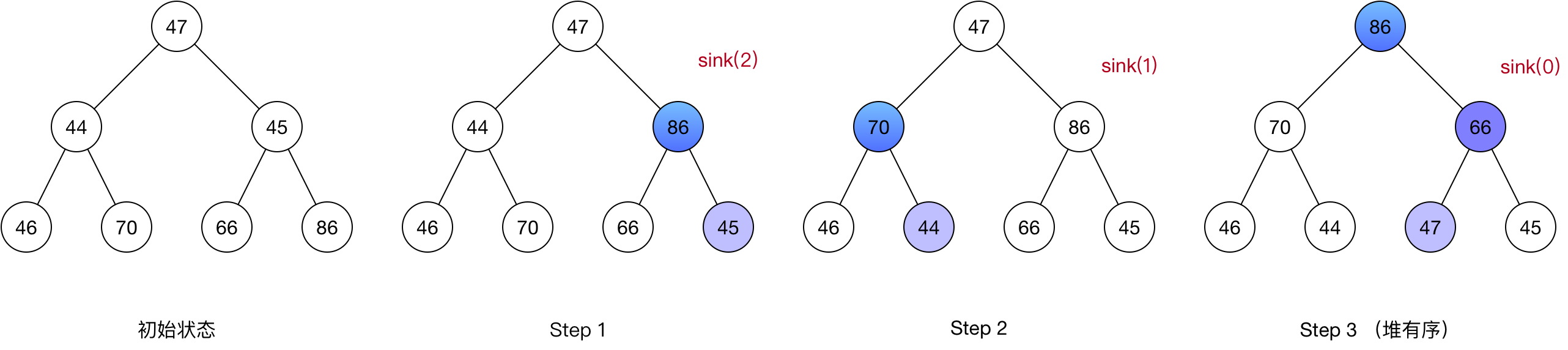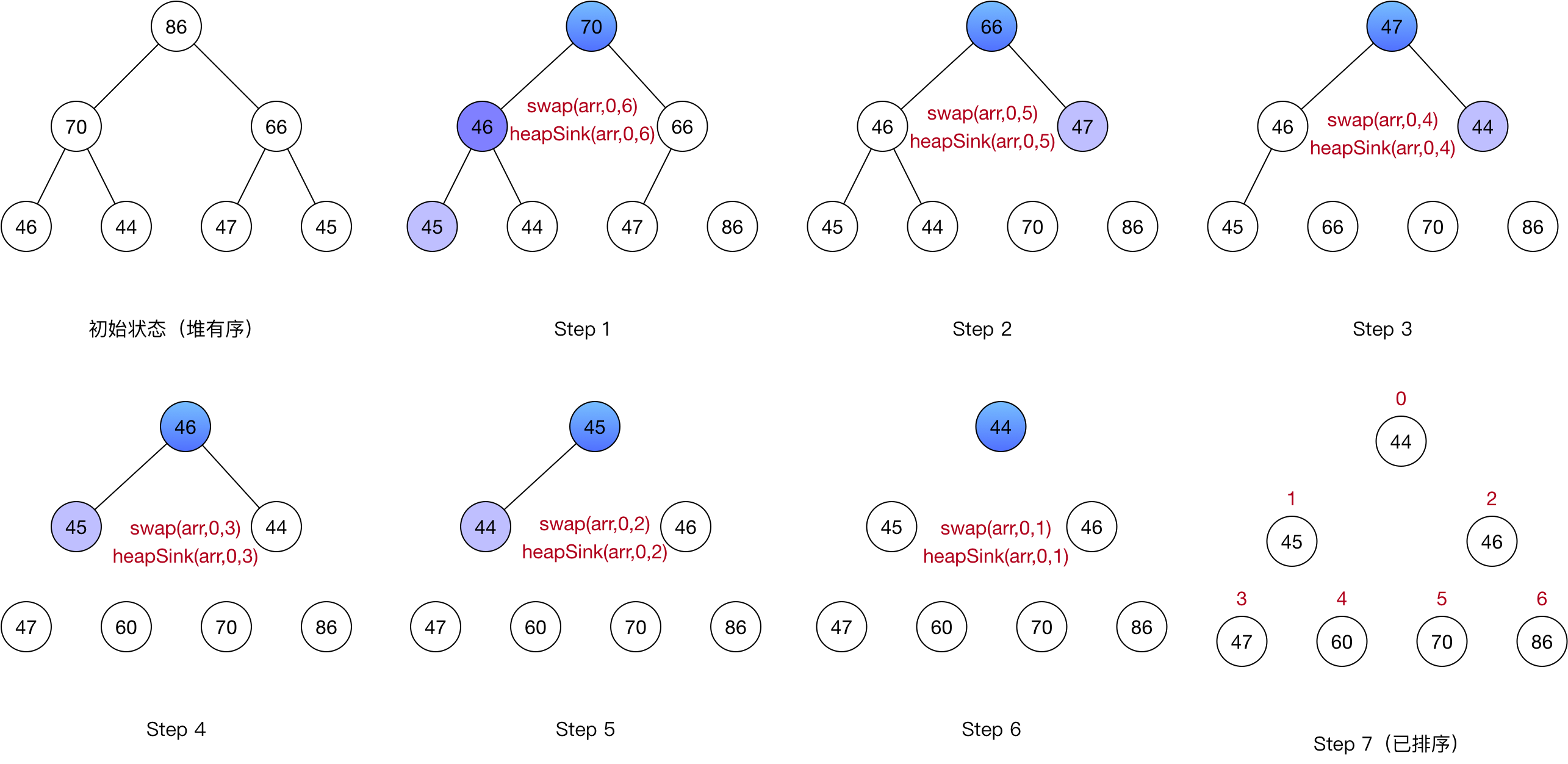### 堆#

1. 每一个父结点的值都比它的子结点大（称为大顶堆）或小（称为小顶堆）
2. 子结点的大小与其左右位置无关

1. 使用指针，二叉树的每个结点需存储三个指针，分别指向其父结点和两个子结点
2. 使用数组，对二叉树做层序遍历，按层级顺序放入数组中，根结点在数组索引0的位置存放，其子结点分别在索引1和2的位置，1和2个子结点分别在位置3、4和5、6中存放，以此类推

### 堆有序化#

1. 在堆底加入一个较大元素时，我们需要由下至上恢复堆的顺序
2. 当将根结点替换为一个较小元素时，我们需要由上到下恢复堆的顺序

#### 由下至上的堆有序化（上浮）#

 ``````1 2 3 4 5 6 `````` ``````private void swim(Integer arr[], int k) { while(k > 0 && arr[(k - 1) / 2] < arr[k]) { //若k>0且索引为k的结点大于其父结点时，将该结点与其父结点交换 swap(arr, k, (k - 1) / 2); k = (k - 1) / 2; } } ``````

#### 由上至下的堆有序化（下沉）#

 `````` 1 2 3 4 5 6 7 8 9 10 11 12 `````` ``````private void sink(Integer arr[], int k) { while(2 * k + 1 <= arr.length - 1) {//若k存在子结点，则进入循环 int j = 2 * k + 1; //获取k的第一个子结点 if (j < arr.length - 1 && arr[j] < arr[j + 1]) {//若存在两个子结点，则找到其中较大的子结点 j++; } if (arr[j] > arr[k]) {//若k的较大子结点比k大，则交换它们的位置 swap(arr, j, k); k = j; } } } ``````

### 堆排序#

#### 堆的构造#

1. 将原数组看做堆的话，则最后一个分支结点（含有子结点的结点）在原数组中的索引为 (n-1)/2 -1
2. 从(n-1)/2-1向前依次执行下沉操作，从而得到堆有序的数组#### 堆的排序#

1. 取出堆的根结点，与数组最后一个元素交换。交换后堆有序状态可能会被打破，需要在新的根结点进行下沉操作，使其恢复为堆有序状态。此时数组中最大(大顶堆)/最小(小顶堆)的值存放在数组末位，除它以外的最 大/小 值位于堆顶。
2. 从数组中排除最后一个元素，重复步骤2，直到数组中的元素全部排除时，完成排序`````` 1 2 3 4 5 6 7 8 9 10 11 12 13 14 15 16 17 18 19 20 21 22 23 24 25 26 27 `````` ``````public static void heapSort(Integer arr[]) { int n = arr.length; //堆的构造,对每一个含有孩子的结点做下沉操作,得到大顶堆 for (int i = (n-1) /2 -1; i >= 0; i--) { heapSink(arr, i, n); } printArr(arr, "大顶堆"); for (int i = n - 1; i > 0; i--) { swap(arr, 0, i); heapSink(arr, 0, i); } printArr(arr, "堆排序"); } public static void heapSink(Integer arr[], int i, int length) { while(2 * k + 1 <= length - 1) {//若k存在子结点，则进入循环 int j = 2 * k + 1; //获取k的第一个子结点 if (j < length - 1 && arr[j] < arr[j + 1]) {//若存在两个子结点，则找到其中较大的子结点 j++; } if (arr[j] > arr[k]) {//若k的较大子结点比k大，则交换它们的位置 swap(arr, j, k); k = j; } } } ``````